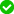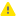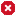# Status Property### Description

Use the `Status` property to determine the status of the log item the LogParamObj object corresponds to.

### Declaration

LogItemObj.Status

 LogItemObj An expression, variable or parameter that specifies a reference to a LogItem object

### Applies To

The property is applied to the following object:

### Property Value

One of the following constants that specify the log item status:

Constant Value Description
`lsOk` 0 The log item contains only informative messages. These log items have theicon.
`lsWarning` 1 The log item contains warning and informative messages and does not contain error messages. In this case, the log item has theicon.
`lsError` 2 The log item contains one or more error messages. Such log items have theicon in the TestComplete IDE.

### Example

The code below obtains a collection of logs that belong to the current project and posts the name and status of each log item to the test log.

JavaScript, JScript

function LogResultsExample()
{
// Obtains a collection of logs
var LogsCol = Project.Logs;
// Obtains the total number of log items in the collection
var Num = LogsCol.LogItemsCount;

if ( Num > 0 )
{
// Iterates through the items
for (var i = 0; i < Num; i++)
{
var LogItem = LogsCol.LogItem(i);
var Name = LogItem.Name;
var Status = LogItem.Status;
Log.Message("The " + Name + " item has the following status: " + Status);
}
}
else
Log.Message("The collection has no log items.");

}

Python

``````def LogResultsExample():
# sObtains a collection of logs
LogsCol = Project.Logs
# Obtains the total number of log items in the collection
Num = LogsCol.LogItemsCount
if Num > 0:
# Iterates through the items
for i in range(0, Num):
LogItem = LogsCol.LogItem[i]
Name = LogItem.Name
Status = LogItem.Status
Log.Message("The " + Name + " item has the following status: " + str(Status))
else:
Log.Message("The collection has no log items.")``````

VBScript

Sub LogResultsExample()

' Obtains a collection of logs
Set LogsCol = Project.Logs
' Obtains the total number of log items in the collection
Num = LogsCol.LogItemsCount

If Num > 0 Then
' Iterates through the items
For i = 0 to (Num - 1)
Set LogItem = LogsCol.LogItem(i)
Name = LogItem.Name
Status = LogItem.Status
Log.Message("The " & Name & " item has the following status: " & Status)
Next
Else
Log.Message("The collection has no log items.")
End If

End Sub

DelphiScript

function LogResultsExample;
var LogsCol, Num, i, LogItem, Name, Status;
begin

// Obtains a collection of logs
LogsCol := Project.Logs;
// Obtains the total number of log items in the collection
Num := LogsCol.LogItemsCount;

if ( Num > 0 ) then
begin
// Iterates through the items
for i := 0 to (Num - 1) do
begin
LogItem := LogsCol.LogItem[i];
Name := LogItem.Name;
Status := LogItem.Status;
Log.Message('The ' + Name + ' item has the following status: ' + Status);
end
end
else
Log.Message('The collection has no log items.');
end;

C++Script, C#Script

function LogResultsExample()
{
// Obtains a collection of logs
var LogsCol = Project["Logs"];
// Obtains the total number of log items in the collection
var Num = LogsCol["LogItemsCount"];

if ( Num > 0 )
{
// Iterates through the items
for (var i = 0; i < Num; i++)
{
var LogItem = LogsCol["LogItem"](i);
var Name = LogItem["Name"];
var Status = LogItem["Status"];
Log["Message"]("The " + Name + " item has the following status: " + Status);
}
}
else
Log["Message"]("The collection has no log items.");

}